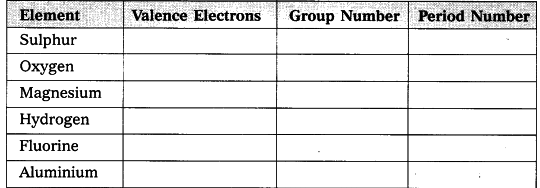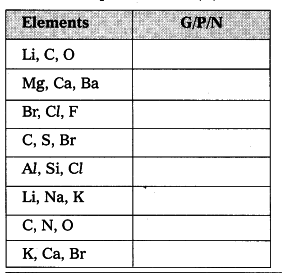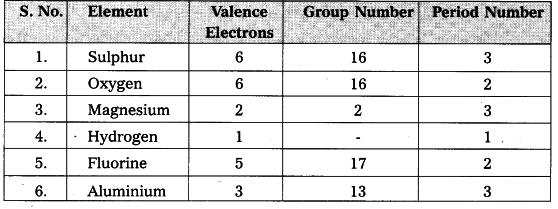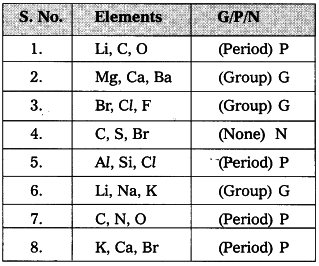# State the number of valence electrons

a) State the number of valence electrons, the group number and the period num¬ber of each element given in the following table.b) State whether the following elements belong to a Group (G), Period § or Neither Group nor Period (N).a)b)